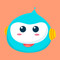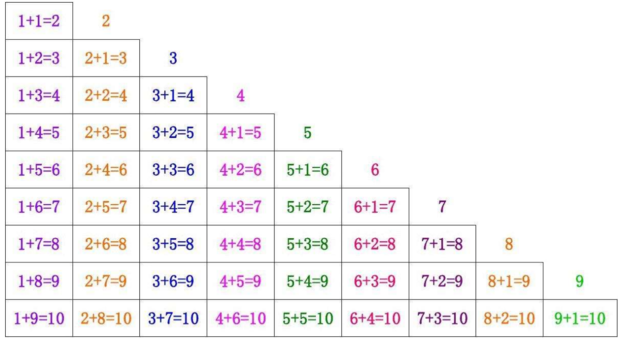KAJKJAKJA LV12 2013-02-23

### 满意答案Dsbabuzl
LV12

10以内加法口诀：

1+1=2 1+2=3 1+3=4 1+4=5 1+5=6 1+6=7 1+7=8 1+8=9 1+9=10

2+1=3 2+2=4 2+3=5 2+4=6 2+5=7 2+6=8 2+7=9 2+8=10

3+1=4 3+2=5 3+3=6 3+4=7 3+5=8 3+6=9 3+7=10

4+1=5 4+2=6 4+3=7 4+4=8 4+5=9 4+6=10

5+1=6 5+2=7 5+3=8 5+4=9 5+5=10

6+1=7 6+2=8 6+3=9 6+4=10

7+1=8 7+2=9 7+3=10

8+1=9 8+2=10

9+1=10

10以内减法口诀：

9-9=0 9-8=1 9-7=2 9-6=3 9-5=4 9-4=5 9-3=6 9-2=7 9-1=8

8-8=0 8-7=1 8-6=2 8-5=3 8-4=4 8-3=5 8-2=6 8-1=7

7-7=0 7-6=1 7-5=2 7-4=3 7-3=4 7-2=5 7-1=6

6-6=0 6-5=1 6-4=2 6-3=3 6-2=4 6-1=5

5-5=0 5-4=1 5-3=2 5-2=1 5-1=4

4-4=0 4-3=1 4-2=2 4-1=3

3-3=0 3-2=1 3-1=2

2-2=0 2-1=1

1-1=01，加法速算：计算任意位数的加法速算，方法很简单学习者只要熟记一种加法速算通用口诀“本位相加（针对进位数） 减加补，前位相加多加一 ”就可以彻底解决任意位数从高位数到低位数的加法速算方法。

2，减法速算：计算任意位数的减法速算方法也同样是用一种减法速算通用口诀 ——“本位相减（针对借位数） 加减补，前位相减多减一 ”就可以彻底解决任意位数从高位数到低位数的减法速算方法。

3，乘法速算：魏氏乘法速算通用公式：ab×cd=(a+1)×c×100+b×d+魏氏速算嬗数×10。

（1），用第一种速算嬗数=（a-c）×d+（b+d-10）×c，适用于首同尾任意的二位数乘法速算，比如 ：26×28, 47×48，87×84-----等等，其嬗数一目了然分别等于“8”，“20 ”和“8”即可。

（2），用第二种速算嬗数=（a+b-10）×c+（d-c）×a适用于一因数的二位数之和接近等于“10”,另一因数的二位数之差接近等于“0”的任意二位数乘法速算 ，比如 ：28×67, 47×98, 73×88----等等 ，其嬗数也同样可以一目了然分别等于“2”，“5 ”和“0”即可。

（3），用第三种速算嬗数=a×d-‘b’（补数）×c 适用于任意二位数的乘法速算。

1413